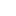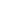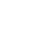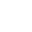Flash Cards

# 【英語】数学の動詞フラッシュカード（Math）Three ＿＿ five ＿＿s eight.
Three ＿＿ one ＿＿s two.
Three ＿＿ two ＿＿s six.
Eight ＿＿ed ＿＿ two ＿＿s four.## 動詞フラッシュカード

#### 音声付きフラッシュカードアバン
3 and 5 equals 8./3 plus 5 equals 8.
Three minus one equals two.
Three times two equals six.
division./Eight divided by two equals four.なぜ日本人は何度も英語をやり直し続けているのか？まだの方は【電子書籍版】でお読み下さい。